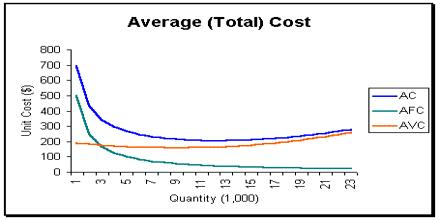Economics

# Average CostThis article talks about Average Cost, which is a cost accounting term that is sometimes referred to as unit cost or weighted average cost. It can refer to either average cost of inventory or the average cost of units produced. It is also equal to the sum of average variable costs plus average fixed costs. It affect the supply curve and are a fundamental component of supply and demand. Average Cost is important to understand that firms maximize profits by considering the marginal cost, not the average cost. Average cost produces results that fall somewhere between FIFO and LIFO.